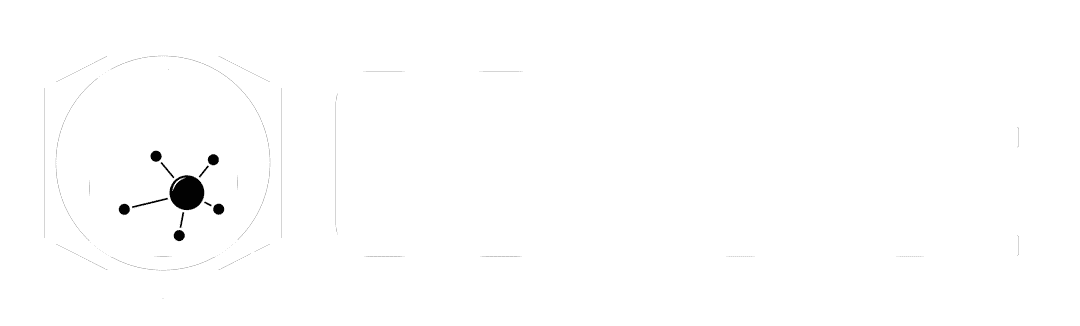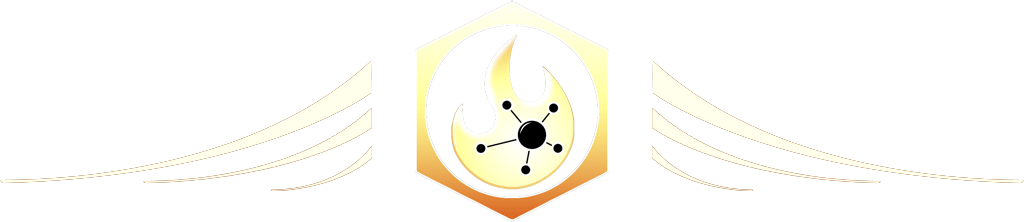# Normal

The Ultimate Houdini node reference

# Visit the Node Bible

### Normal

The Normal node will generate normals for points, primitives, or vertices based off of the winding order of the primitive.  In order to see what this does, make sure to watch the videos above.
Drag to resize

Summary:

In 3D, the "normal" of something typically refers to the direction that a point, vertex, or primitive is facing.  Most often, this is very applicable to the primitives (polygons) in your scene.  Polygons need to be facing the correct direction to render properly.  Plus, there are many situations in VFX which will rely on this information being accurately described.  The normal of the polygon (which is a primitive) is determined by the winding order of a primitive.  The winding order describes how the polygon was made, and the direction in which the vertices are drawn is essentially this winding order.  This direction can then be used to determine the normal of the polygon.

If you are noticing that the Normal node is not fixing your normals, then it's probably because the winding order of your primitives are incorrect.

To fix the winding order, you can use the reverse sop, or you can try using a setting on the poly doctor node and going under Topology --> Correct Winding of Polygons to Majority in their Manifold Patch.  Once a good winding order is established, you can then run this normal sop to establish proper normals.

Drag to resize

General Parameters:

Group + Group Type:

--  This specifies where the normal node will operate along your mesh.  For more info on groups, please visit the Group Node Bible entry.

Override Normal:

--  This parameter can be used to affect an attribute other than N (which is used for normals be default in Houdini).  In practice, this can be useful for specifying other vector attributes (such as v for velocity).

Compute Normals:

--  This toggle will re-calculate the normals.  The only situation which requires turning this off is when you want to modify the existing normal data.  Specifically, you can either reverse the direction or "Make Normals Unit Length" to existing normals if you'd like.

--  Vertices = By default, it will assign this to vertices.  For more information about the difference between points, vertices, and primitives, please watch the video above.  When setting normal data to your vertices, it's typically for shading purposes when rendering.  Vertex normals are great because they offer an accurate way of describing the smoothing between faces.  Because each vertex is associated with a specific primitive, this means that there are typically 4 vertices at each point facing different directions (according to the associated primitive).  Because of this, it allows the render engine to produce a higher quality smoothing than would would otherwise be possible with just one point or primitive normal.

--  Points = Setting point normals is not intended for rendering purposes.  However, it is very useful for a variety of FX tasks which may rely on this vector information to drive certain effects.  So, if you're trying to use Normal information for purposes other than rendering, then this may be the setting you're looking for.

--  Primitives =  This sets a normal that's associated with the primitive instead of the point.  Again, this is typically used for FX-related tasks.

--  According to the docs, "A single normal will be computed for the detail, being the average of the primitive normals, weighted by the primitive area."  In practice, this might be useful for determining the overall direction that something is facing.

Cusp Angle:

--  This parameter gets used to determine whether or not the viewport (or render engine) will smooth the normals of the mesh so that they don't look faceted (blocky).  According to the docs, "When computing vertex normals, if normals of vertices around a single point are less than or equal to this angle (in degrees) apart from each other, they will be averaged together, weighted by the vertex angle in each polygon."  If you are adjusting point or prim normals, then keep an eye on how this parameter will alter the direction of the normals.

Weighting Method:

--  These settings determine how the normals get smoothed out when adjusting the cusp angle.  According to the docs, "When set to By Vertex Angle, care is taken when computing normals to try to avoid issues introduced by sliver polygons and to weight contributions to normals by angle, so that triangulation won’t change normals significantly. When set to Each Vertex Equally, a faster, but less accurate, approach similar to the approach used by the Facet SOP, is used to compute normals, where each vertex is given equal weight around a point, and less checks are done for ensuring stability. When set to By Face Area, the behavior is similar to __Each Vertex Equally_, except that vertices on faces with more area will be given more weight when computing point normals. This is often used to fake the appearance of a smooth bevel for geometry with only a simple chamfer."  In practice, the "By Vertex Angle" should be used for most situations unless you are trying to model and render with simple chamfer bevels.

Keep Original Normal Where the Computed Normal Is Zero:

--  This setting can be important to consider when calculating normals that get used for FX purposes.  If you have floating points that are not associated with any vertex/primitive, then this will take any existing normal information and set it to 0,0,0.  This is especially important to consider if you're trying to use this node to affect velocity instead of normals.  In most situations, I would suggest leaving this off unless you're trying to reset those attributes.

Modify:

Make Normals Unit Length:

--  This will make sure that the resulting normals are "normalized."  (I know, that's kind of confusing)  When something is "normalized" it means that the values exist between 0 and 1.  So, if a normal value is something crazy like (5,1,1), "normalizing" it will cause each element of that vector to exist between 0 and 1.  So, the new value would be (1, 0.2, 0.2)

Reverse Normals:

--  This will reverse the direction of the calculated normal vector.  This will NOT fix the winding order of a primitive if it is broken.  For more info on this, please read the summary section above and/or watch the videos.

Drag to resize